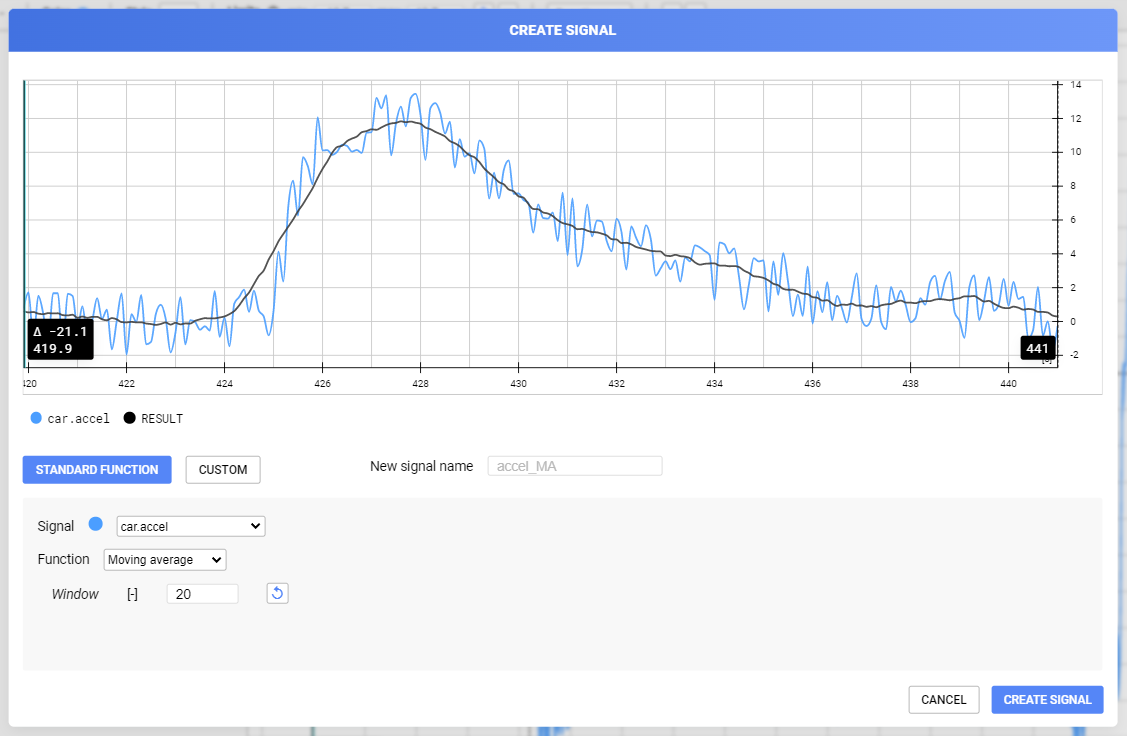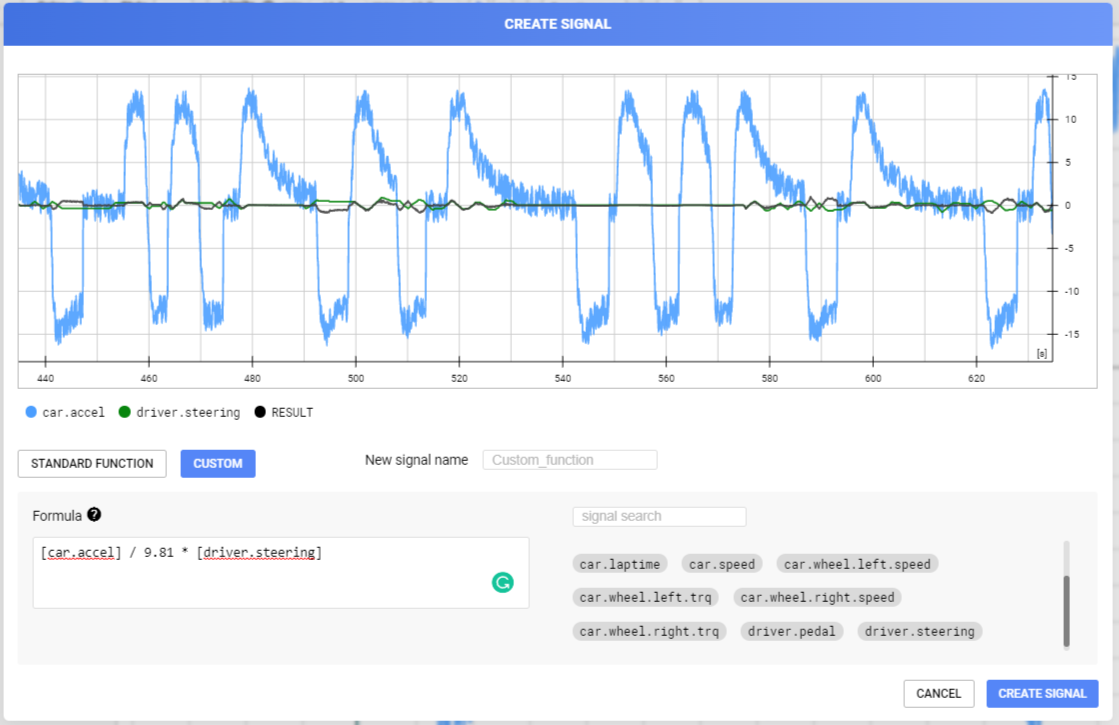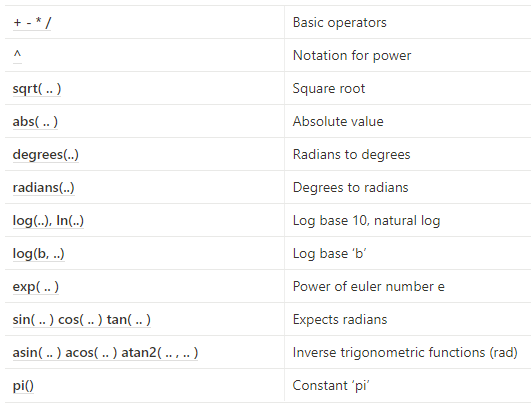# Calculated Signals

Create new signals by applying filters or combining different signals.
There are two types of calculations you can perform on signals:
• Standard functions
• Custom functions

## Standard Functions

To create a standard function, select the signal on which you want to do a calculation, hit the 'Functions' button and you should see a preview window appear.There are multiple standard functions, a detailed explanation can be found here:
Moving Average
Central moving average that takes the average of (window / 2) samples to the left, the current sample and (window / 2 - 1) samples to the right.
Parameters:
• Window
Cumulative sum
Calculates the sum of all samples to the left and the current sample.
Parameters:
• Gain
Derivative
Calculates the backwards discrete derivative of the signal.
d[k] = (v[k] - v[k-1]) / (t[k] - t[k-1])
Parameters:
• Gain
Difference
Calculates the backwards difference of the signal.
Calibration
Calculates a simple calibration for a signal.
c = (v + offset) * scale
Parameters:
• scale
• offset
Remove Outliers (custom)
Removes outliers from a signal. All values outside the bounds will be removed.
Parameters:
• max bound
• min bound
Remove Outliers (stddev)
Removes outliers from a signal: all values outside of [avg(s) - x * σ, avg(s) + x * σ] will be removed.
Parameters:
• factor of standard deviation to define outliers
Clamping
Clamps a signal. All values outside the bounds will be clamped to the bound.
Parameters:
• max bound
• min bound
Zeroing
Zeroing removes the bias of a signal by subtracting the first value of the signal of all the data points.
Sample shift
Shift the entire signal with a few samples.
Parameters:
• offset
‍‍

## Custom functions

Standard functions let you create some more complex functions on data, but sometimes you just need to make a custom function where you add, subtract, .. some signals with each other. The custom functions are the place to be.
To create a custom function press the create signal button in the signal sidebar, this should by default switch to the custom function modal.
Note that in your formula, make sure the signal names are between brackets. To make things easier you can search signals on the right side and click the signal names to add them in the formula.#### Supported OperatorsConditional Statements
Case statements allow even more flexibility, and can be used wherever an expression is valid.
CASE WHEN condition THEN result [WHEN ...] [ELSE result] END

#### Examples

[sig_a]^2 + log([sig_b])/[sig_c_LP]
abs(sin([sig_a] * pi()))
case when [C.vx] > 21 then 1 else 0 end